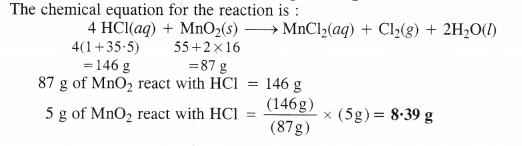## NCERT Solutions for Class 11 Chemistry Chapter 1 Some Basic Concepts of Chemistry

These Solutions are part of NCERT Solutions for Class 11 Chemistry. Here we have given NCERT Solutions for Class 11 Chemistry Chapter 1 Some Basic Concepts of Chemistry.

Question 1.
Calculate the molecular mass of the following :
(i) H2O (ii) CO2 (iii) CH4
(i) Molecular mass of H2O : 2 × 1 + 1 × 16 = 18u
(ii) Molecular mass of CO2 : 1 × 12 + 2× 16 = 44 u
(iii) Molecular mass of CH4 : 12 + 4 × 1 = 16 u

Question 2.
Calculate the mass percent of different elements present in sodium sulphate (Na2SO4).
Molecular mass of Na2SO4 = 2 × Atomic mass of Na + Atomic mass of S + 4 × Atomic mass of O
= 2 × 23 + 32 + 4 × 16 = 46 + 32 + 64 = 142 u.
The percentage of different elements present can be calculated as :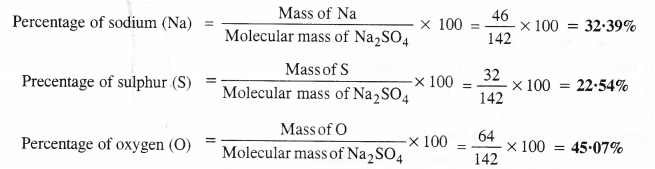Question 3.
Determine the empirical formula of an oxide of iron which has 69-9% iron and 30-1% oxygen by mass.
Step I. Calculation of simplest whole number ratios of the elements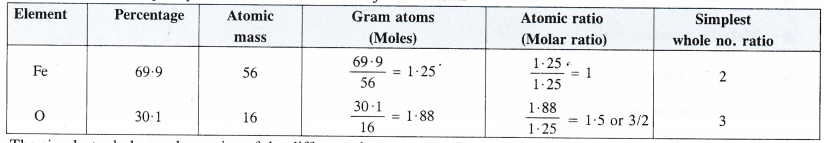The simplest whole number ratios of the different elements are : Fe : O : : 2 : 3
Step II. Writing the empirical formula of the compound.
The empirical formula of the compound = Fe2CO3.

Question 4.
Calculate the amount of carbon dioxide that could be produced when
(i) 1 mole of carbon is burnt in air.
(ii) 1 mole of carbon is burnt in 16 g of dioxygen.
(iii) 2 moles of carbon are burnt in 16 g of dioxygen.
The chemical equation for the combustion of carbon in dioxygen present in air is :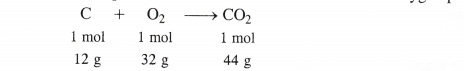(i) When 1 mole of carbon is burnt in air
1 mole of carbon will form C02 = 1 mol = 44 g
(ii) When 1 mole of carbon is burnt in 16 g of dioxygen
For 1 mole of carbon, dioxygen required = 32 g = 1 mol
But the mass of dioxygen available = 16 g = 1/2 mol .
This means that dioxygen is in limited amount or-it is the limiting reactant.
Since dioxygen and carbon react in the same ratio, therefore mass of CO2 formed = $$\frac { 1 }{ 2 }$$ mol = 22 g
(iii) When 2 moles of carbon are burnt in 16 g of dioxygen
For 2 moles of carbon, dioxygen required = 64 g = 2 mol
But the mass of dioxygen available = 16 g =  $$\frac { 1 }{ 2 }$$ mol
This means that dioxygen is in limited amount or it is the limiting reactant.
∴ Mass of C02 formed =$$\frac { 1 }{ 2 }$$ mol = 22 g

Question 5.
Calculate the mass of sodium acetate (CH3COONa) required to make 500 mL of 0-375 molar aqueous solution. Molar mass of sodium acetate is 82.0 g mol-1.Question 6.
Calculate the concentration of nitric acid in moles per liter in a sample which has a density of 1.41 g mL-1 and the mass percent of nitric acid in it being 69%.
Mass percent 69 means that 69 g of HNo3 are dissolved in 100 g of the solution.
Mass of solution = 100 g Density of solution = 1.41 g mL-1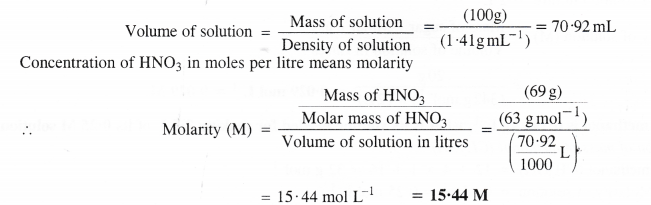Question 7.
How much copper can be obtained from 100 g of copper sulphate (CuSO4)?
The molecular mass of CuSO4 = Atomic mass of Cu + Atomic mass of S + 4 x Atomic mass of O
= 63.5 + 32 + 4 × 16
= 159.5 u
Gram molecular mass of CuSO4 = 159.5 g
Now, 159-5 g of CuSO4 have Cu = 63.5 g
∴ 100 g of CuSO4 have Cu = (63.5 g) × $$\frac { (100 g) }{ (159.5 g) }$$ = 39.81 g

Question 8.
Determine the molecular formula of an oxide of iron in which the mass percent of iron and oxygen are 69.9 and 30.1 respectively.
The empirical formula of the oxide of iron = Fe2O3
(For details, refer to No. 3)
Molecular formula of the oxide of iron = n × Empirical formula
= 1 × (Fe2O3)
= Fe2O3
(Since there is no common factor in Fe2O3, therefore n = 1).

Question 9.
Calculate the average atomic mass of chlorine from the following data: Isotope % Natural Abundance Atomic mass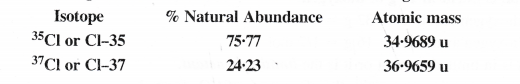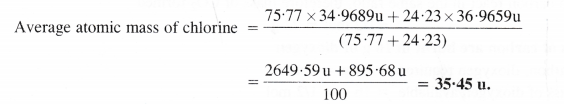Question 10.
In three moles of ethane (C2H6), calculate the following:
(i) No. of moles of carbon atoms
(ii) No. of moles of hydrogen atoms No. of molecules of ethane.
(i) 1 mole of C2H6 has moles of carbon atoms= 2 moles
3 moles of C2H6 have moles of carbon atoms= 2 × 3 = 6 moles
(ii) 1 mole of C2H6 has moles of hydrogen atoms = 6 moles
3 moles of C2H6 have moles of hydrogen atoms = 6 × 3 = 18 moles
(iii) 1 mole of C2H6 has molecules = 6.022 × 1023
3 moles of C2H6 have molecules = 6.022 × 1023 x 3 = 1.81 x 1024

Question 11.
What is the concentration of sugar (C12H22O11) in mol L-1 of it are dissolved in enough water to make final volume upto 2 L?
The concentration in mol L-1 means molarity (M).
From the available data, it can be calculated as:
Mass of sugar = 20 g
Molar mass of sugar (C12H22o11) = 12 × 12 + 22 × 1 + 11 × 16 = 342 g mol-1
Volume of solution in litre = 2 L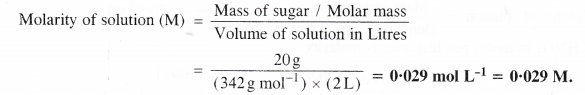Question 12.
If the density of methanol is 0.793 kg L-1, what is its volume needed for making 2.5 L of its 0.25 M solution?
Step I. Calculation of the mass of methanol (CH3OH)
Molar mass of methanol (CH3OH) =12 + 4×1 + 16 = 32 g mol-1
Molarity of solution = 0.25 M = 0.25 mol L-1
Volume of solution = 2.5 LStep II. Calculation of volume of methanol
Mass of methanol = 20 g = 0-002 kg
Density of methanol = 0-793 kg L-1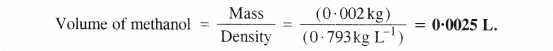Question 13.
The pressure is determined as force per unit area of the surface. The SI unit of pressure, pascal is as shown below:
IPa = 1 Nm-2
If the mass of air at sea level is 1034 g, calculate the pressure in pascal.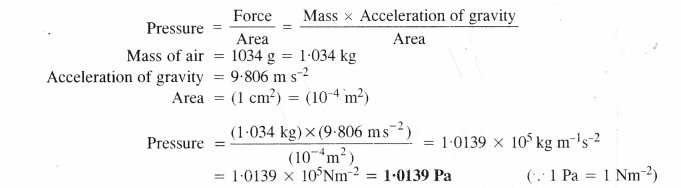Question 14.
What is the SI unit of mass? How is it defined?
Kilogram. It is equal to the mass of the prototype of the kilogram. It is in fact, the mass of a platinum block stored at the International Bureau of Weights and Measurements in France.

Question 15.
Match the following prefixes with their multiples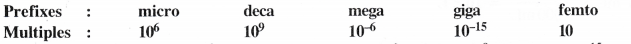After matching:
micro = 10-6
deca = 10
mega = 106
giga = 109
femto = 10-15

Question 16.
What do you understand by significant figures?
We have seen that every measurement done in the laboratory involves the same error or uncertainty depending upon the limitation of the measuring instrument. In order to report scientific data, the term ‘significant figures’ has been used. According to this, all digits repotted in a given data are certain except the last one which is uncertain or doubtful. For example, let us suppose that the reading as reported by a measuring scale is 11-64. It has four digits in all. Out of the 1, 1, and 6 are certain digits while the last digit ‘4’ is uncertain. Thus, the number may be reported as follows :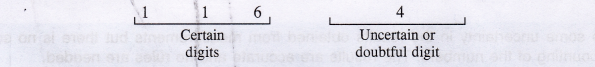Thus, the significant figures in any number are all certain digits plus one doubtful digit.
It may be noted all digits reported in a number are significant. However, only the last digit is uncertain while the rest are certain. Thus, the number 11-64 has all four digits as significant figures. Out of the 1, 1 and 6 are certain while 4 has some uncertainty about it.

Question 17.
A sample of drinking water was found to be severely contaminated with chloroform CHCI3, supposed to be a carcinogen. The level of contamination was 15 ppm (by mass)
(i) Express this in percent by mass.
(ii) Determine the molality of chloroform in the water sample.
(i) Calculation of percent by mass
15 ppm level of contamination means that 15 parts or 15 g of chloroform (CHCI3) are present in 106 parts or 106 g of the sample i.e., water.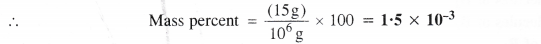(ii) Calculation of molality of the solution
Mass of chloroform = 1.5 x 10-3 g
Molar mass of chloroform (CHCI3) = 12 + 1 + (3 × 35.5) = 119.5 g mol-1
Mass of sample i.e., water = 100 g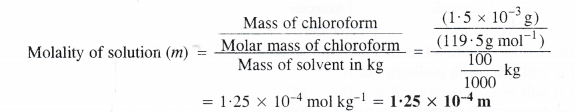Question 18.
following in the scientific notation:
(i) 0.0048
(ii) 234,000
(iii) 8008
(iv) 500.0
(v) 6.0012

(i) 4.8 x 10-3
(ii) 2.34 × 105
(iii) 8.008 × 103
(iv) 5.000 × 102
(v) 6.0012 × 100

Question 19.
How many significant figures are present in the following?
(i) 0.0025
(ii) 208
(iii) 5005
(iv) 126,000
(iv) 500.0.
(v) 2.0034

(i) 2
(ii) 3
(iii) 4
(iv) 6
(v) 4
(vi) 5

Question 20.
Round up the following upto three significant figures :
(i) 34.216
(ii) 10.4107
(iii) 0.04597
(iv) 2808

(i) 34.2
(ii) 10.4
(iii) 0.0460
(iv) 281

Question 21a.
The following data is obtained when dinitrogen and dioxygen react together to form different compounds :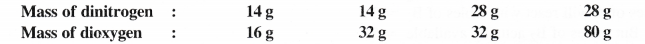By keeping 14 g as the fixed mass of dinitrogen (N2), the ratios by mass of dioxygen (O2) combining with 14 g dinitrogen are : 16 : 32 : 16 : 40 or 2 : 4 : 2 : 5. Since this ratio is simple whole number, the data obeys the Law Multiple Proportions.

Question 21b.
Fill in the blanks in the following conversions :
(i) 1 km = ……… mm = ……….. pm
(ii) 1 mg = …….. kg = …………. ng
(iii) 1 mL = ……. L = ………… dm3.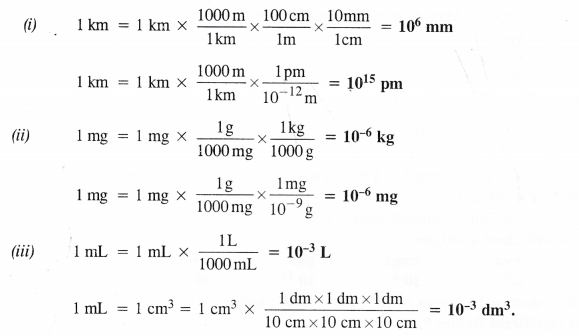Question 22.
If the speed of light is 3.0 x 108 m s-1, calculate the distance covered by light in 2.00 ns.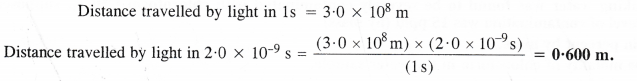Question 23.
Identify the limiting reactant if any in the following reaction mixtures ?
A + B → AB2
(i) 300 atoms of A + 200 molecules of B2
(ii) 100 atoms of A + 100 molecules of B2
(iii) 5 moles of A + 2.5 moles of B2
(iv) 2.5 moles of A + 5 moles of B2
(v) 2 moles of A + 3 moles of B2.In the light of the above information, let us find the limiting reactant if any in all the cases
(i) 1 atom of A will react with molecules of B2 =1
300 atoms of A will react with molecules of B2 = 300
But the molecules of B2 actually available = 200
∴B2 is the limiting reactant.

(ii) 1 atom of A will react with molecules of B2 = 1
100 atoms of A will react with molecules of B2 = 100
The molecules of B2 actually available = 100
∴There is no limiting reactant in this case.

(iii) 1 mole of A will react with moles of B2 =1
5 moles of A will react with moles of B2 =5
But the moles of B2 actually available = 2.5
∴B2 is the limiting reactant.

(iv) 1 mole of A will react with moles of B2 =1
2.5 moles of A will react with moles of B2 = 2.5
But moles of B2 actually available = 5
This shows that 5 moles of A can react whereas only 2.5 moles of A are actually available.
∴A is the limiting reactant.

(v) 1 mole of A will react with moles of B2 = 1
2 moles of A will react with moles of B = 2
But the moles of B2 actually available = 3
This shows that 3 moles of A can react whereas only 2 moles of A are actually available.
∴ A is the limiting reactant.

Question 24.
Nitrogen and hydrogen react to form ammonia according to the reaction
N2 (g) + 3H2 (g) →2NH3(g)
If 1000 g of H2 react with 2000 g of N2,
(i) will any of the two reactants remain unreacted ? If yes, which one and what would be its mass ?
(ii) Calculate the mass of ammonia (NH3) which will be formed.According to available data,
28 g of N2 require H2 = 6 g
2000 g of N2 require H2 = (6g) x $$\frac { 2000 g }{ 28 g }$$=428.6 g
But H2 actually available = 1000 g
This means that H2 is in excess and will remain unreacted.
(i) Mass of H2 that remains unreacted = 1000 – 428.6 = 571.4 g
(ii) Mass of NH3 formed may be calculated as follows :
6 g of H2 will form NH3 = 34 g
428.6 g H2 will form NH3 = (34g) x $$\frac { 428.6 g }{ 6.0 g }$$ = 2428.8 g

Question 25.
How are 0.50 mol Na2Co3 and 0.50 M Na2Co3 different ?
0.50 mol Na2CO3 represent concentration in moles.
0.50 M Na2CO3 represent concentration in moles/litre (molarity).

Question 26.
If 10 volumes of dihydrogen react with five volumes of dioxygen gas, how many volumes of water will be produced ?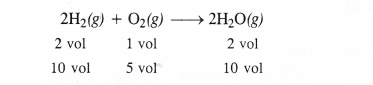10 volumes of water vapours will be produced.

Question 27.
Convert the following into basic units
(i) 28.7 pm
(ii) 15.15 \xs
(iii) 25365 mg.Question 28.
Which of the following has largest number of atoms ?
(i) 1 g of Au
(ii) lg of Na
(iii) 1 g of Li
(iv) lg of Cl2

(i) 197 g of Au have atoms = 6.022 x 1023
∴ l g of Au has atoms = 6.022 × 1023 × $$\frac { 1 g }{ 197g }$$ = 3.06 x 1021
(ii) 23 g of Na have atoms = 6.022 × 1023
1 g of Na has atoms = 6 -022 × 1023 × $$\frac { 1 g }{ 23g }$$ = 2.62 x 1022 atoms
(iii) 71 g of Cl2 have molecules = 6.022 × 1023
71 g of Cl2 have atoms = 2 × 6.022 x 1023
1 g of Cl2 has atoms = 2 × 6.022 × 1023 x $$\frac { 1 g }{ 71g }$$ = 1.67 × 1022 atoms
Thus, 1 g of lithium (Li) has the largest number of atoms.

Question 29.
Calculate the molarity of a solution of ethanol in water in which mole fraction of ethanol is 0.04.2.31 moles of ethanol are dissolved in 1000 g (or 1000 mL) of water or 1000 mL of the solution. In this case, the volume of solution is considered to be the same as that of the solvent i.e., water. In other words, the solution is regarded as dilute solution,
∴Molarity of solution = 2.31 M

Question 30.
What will be mass of one 12C in g ?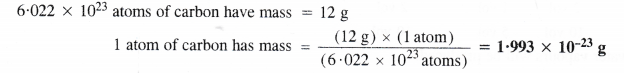Question 31.
How many significant figures should be present in the answer of the following calculations ?
(i) $$\frac { 0.2856 x 298.15 x 0.112 }{ 0.5785 }$$
(ii) 5 x 5.364
(iii) 0.0125 + 0-7864 4- 0.0215.

(i) The least precise figure (0.112) has 3 significant figures. Therefore, the answer should have three significant figures.
(ii) The second figure (5.364) has 4 significant figures. Therefore, the answer should be reported upto four significant figures. The exact figure (5) is not considered in this case.
(iii) In this case, the least precise figures (0.0125 and 0.0215) have 3 significant figures. Therefore, the answer should be reported upto three significant figures.

Question 32.
Use the data given in the following table to calculate the molar mass of naturally occurring argon.Molar mass of argon is the average molar mass and may be calculated as :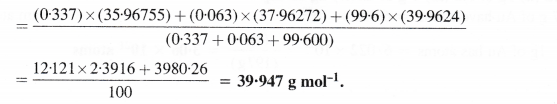Question 33.
Calculate the number of atoms present in : (i) 52 moles of He (ii) 52 u of He (iii) 52 g of He.
(i) 1 mole of He contains atoms = 6.022 × 1023
52 moles of He contain atoms = 6.022 x 1023 x 52 = 3-13 × 1025 atoms
(ii) Atomic mass of He = 4 u ; 4 u is the mass of He atoms = 1
52 u is the mass of He atoms = $$\frac { 1 }{ 4 }$$ x 52 = 13 atoms
(iii) Gram atomic mass of He = 4 g ; 4 g of He contain atoms = 6.022 × 1023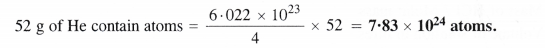Question 34.
A welding fuel gas contains carbon and hydrogen only. Burning a small sample of it in oxygen gives 3.38 g carbon dioxide, 0.690 g water and no other products. A volume of 10.0 L (measured at NTP) of this welding gas is found to weigh 11.6 g. Calculate (i) empirical formula (ii) molar mass and (iii) molecular formula of the gas.
Step I. Calculation of mass percent of carbon and hydrogen.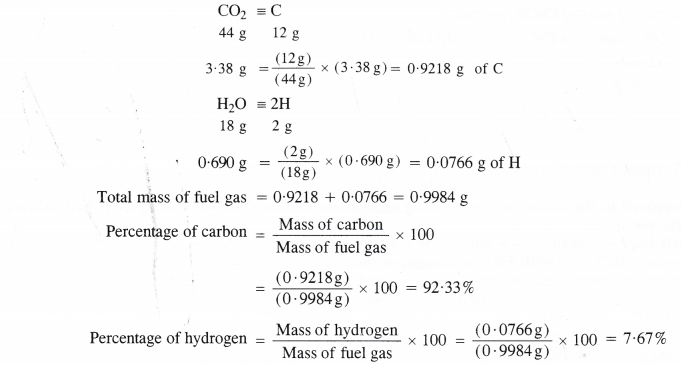Step II. Determination of empirical formula of fuel gas.Empirical formula of the fuel gas = CH.

Step III. Calculation of molecular mass of fuel gas.
10.0 L of the fuel gas at N.T.P. weigh = 11.6 g
22.4 L of the fuel gas at N.T.P. weigh =$$\frac { 11.6 }{ 10.0}$$ × 24.4 =25.98 g
Molecular mass of the fuel gas = 25.98 g $$\approx$$ 26.0 g = 26 u

Step IV. Calculation of molecular formula of the gas.
Empirical formula mass = 12 + 1 = 13 u
Molecular mass = 26 u
n = $$\frac { Molecular mass }{ Empirical formula mass }$$ × $$\frac { 126 }{ 13}$$ = 2
∴ Molecular formula = n × Empirical formula = 2 × CH = C2H2
The molecular formula of fuel gas is C2H2 and it is acetylene.

Question .35.
Calcium carbonate reacts with aqueous HCl to give CaCl2 and Co2 according to the reaction :
CaCo3 (s) + 2HCl (aq) + CaCl2 (aq) + Co2 (g) + H2o (l)
What mass of CaCo3 is required to react completely with 25 mL of 0.75 M HCl ?